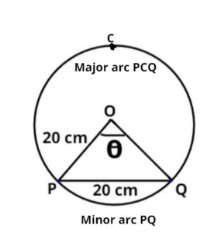Courses
Courses for Kids
Free study material
Free LIVE classes
More# In a circle of diameter 40 cm, the length of a chord is 20 cm. Find the length of the minor arc of the chord.Verified
329.4k+ views
Hint – In a circle of radius r unit, if an arc of length A unit subtends an angle $\theta$ radian at the center, then $\theta = \dfrac{A}{r}$, use this property to reach the solution of the question. Where A = minor arc PQ see figure.Given data
Diameter (D) of the circle = 40 cm
Therefore radius (r) of the circle $= \dfrac{D}{2} = \dfrac{{40}}{2} = 20cm$
Let PQ be a chord of the circle, whose center is O
PQ = 20 cm (given)
In triangle OPQ, OP = OQ = radius of the circle = 20cm
Therefore OP = OQ = PQ = 20 cm
Therefore the triangle is an equilateral triangle.
And we know that in an equilateral triangle all angles are equal $= {60^0}$.
Let the arc of length A unit subtend an angle $\theta$ radian at the center.
Where A = minor arc PQ see figure.
$\Rightarrow \theta = {60^0} = \dfrac{\pi }{3}$ radian.
Now we know that In a circle of radius r unit, if an arc of length A unit subtends an angle $\theta$ radian at the center, then $\theta = \dfrac{A}{r}$, where A is the length of the minor arc PQ.
$\Rightarrow \dfrac{\pi }{3} = \dfrac{A}{{20}}$
$\therefore A = \dfrac{{20}}{3}\pi$ cm.
So the length of the minor arc of the chord PQ be $\dfrac{{20}}{3}\pi$ cm.

Note – In such types of questions first find out the value of angle subtended at the center by the chord of the circle, then according to property of arc which is stated above substitute the values and simplify, we will get the required length of the minor arc of the chord PQ.
Last updated date: 02nd Jun 2023
Total views: 329.4k
Views today: 3.86k